# 基于K阈值的谱聚类算法Spectral Clustering Algorithm Based on K-Threshold

• 全文下载: PDF(2484KB)    PP.969-984   DOI: 10.12677/CSA.2019.95110
• 下载量: 319  浏览量: 503

In the traditional NJW spectral clustering algorithm, similarity measurement between samples is determined by Euclidean distance and Gaussian kernel function. Euclidean distance is based on the whole sample set, ignoring the correlation between local samples. Moreover, the value of Gaussian kernel function is also obtained through multiple experiments, which is easy to fall into local optimization and greatly affects the clustering results. Aiming at the above problems, a new spectral clustering algorithm is proposed, which introduces the concepts of K neighborhood, shortest path and standard deviation. The parameters of similarity measurement between samples are reconstructed, and the relationship between samples is reconstructed with sample standard deviation, so as to modify the value of Gaussian kernel function and make it closer to the characteristics of samples. Through the experimental analysis, the algorithm not only inherits the convergence of spectral clustering algorithm to the whole world, and is applicable to data of various shapes, but also overcomes the limitations of similarity measurement caused by Euclidean distance and Gaussian kernel function, improves the accuracy of the algorithm, and enhances the reliability of clustering results.

1. 引言

2. 谱聚类算法

1) 由输入样本集合X计算样本间欧氏距离Dd$D=\left\{{d}_{ij}\right\}$ ；dij为样本Xi与Xj之间的欧氏距离，由公式(2)可得；

2) 相似度矩阵 $S=\left\{{S}_{ij}\right\}$ ，可以根据给定的公式(1)计算得到；D为度矩阵，是由相似矩阵S得到的对角阵，同时由公式(3)得到拉普拉斯矩阵L；

3) 选出L矩阵中的前C个最大特征值所对应的特征向量，构成新的特征向量空间Z；

4) 用K-means算法对特征向量空间Z进行聚类，得到聚类结果Y。

${d}_{ij}=\sqrt{{\left({x}_{i1}-{x}_{j1}\right)}^{2}+{\left({x}_{i2}-{x}_{j2}\right)}^{2}+\cdots +{\left({x}_{im}-{x}_{jm}\right)}^{2}}$ (1)

${S}_{ij}=\mathrm{exp}\left(-\frac{{‖{x}_{i}-{x}_{j}‖}^{2}}{2{\sigma }^{2}}\right)$ (2)

$L={D}^{-\frac{1}{2}}S{D}^{-\frac{1}{2}}$ (3)

3. NEW-SC谱聚类算法

1) 由输入样本集合X计算样本间欧氏距离D； $D=\left\{{d}_{ij}\right\}$ ；dij为样本Xi与Xj之间的欧氏距离，由公式(2)可得；

2) 根据给定的K值，对每个样本的K近邻样本集合取标准差Bi

3) 对每个样本Xi以其对应的标准差Bi作为阈值，并保留阈值内的样本集合；此时，每个样本Xi中保留的样本数量与样本集本身性质和K值有关；

4) 每个样本仅在其阈值范围内保留了其与其他样本的距离度量，与阈值之外设为样本间距离是间接获得的，即：利用最短距离方法来保持样本集可以成为一个完整的连通图；

5) 用3中得到的标准差Bi，代替相似性度量中的高斯核函数，消除核函数的取值难问题；

6) 于样本Xi在阈值K中的近邻样本数目为Ni，Di是阈值K中的近邻样本集到Xi的距离均值；而于所有样本集X，在其阈值K中的近邻样本数目为N，其中 $N={N}_{1}+{N}_{2}+\cdots +{N}_{n}$ ；D是阈值K中的近邻样本集到分别到其对应的Xi的距离均值；权重值是Q = D/Di是对局部距离和全局距离的统一调整参数，通过每次建立局部与整体的关系，将所有局部距离建立起相关性，使得相似性度量更具有全局性；

7) 把上述各变量带入为相似度计算公式(4)得相似性矩阵S；

8) 将S矩阵带回谱聚类算法(NJW)的第2步后继续执行后续实验步骤；

${S}_{ij}=\mathrm{exp}\left(-\frac{{‖{x}_{i}-{x}_{j}‖}^{2}}{2\ast {B}_{i}{B}_{j}}Q\right)$ (4)

4. 实验

4.1. 实验数据集

4.2. 性能测量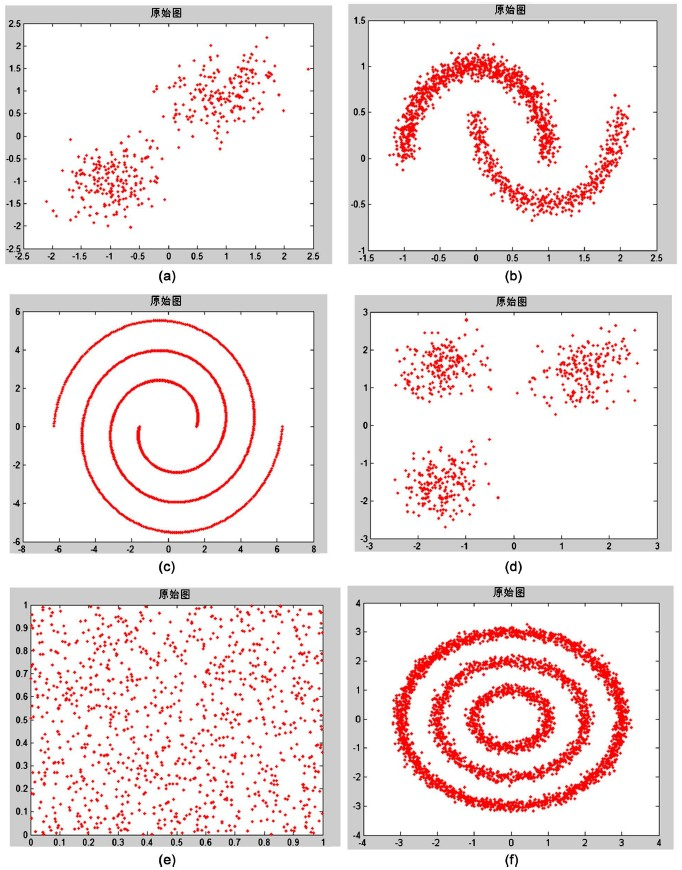Figure 1. Artificial data set information graph: (a) Two-cluster; (b) Two moons; (c) Spiral; (d) Three-circles; (e) Checker-board; (f) Three-cluster

$ACC=\frac{\underset{i=1}{\overset{n}{\sum }}\delta \left({C}_{i},map\left({{C}^{\prime }}_{i}\right)\right)}{n}$ (6)

4.3. 人工数据集实验结果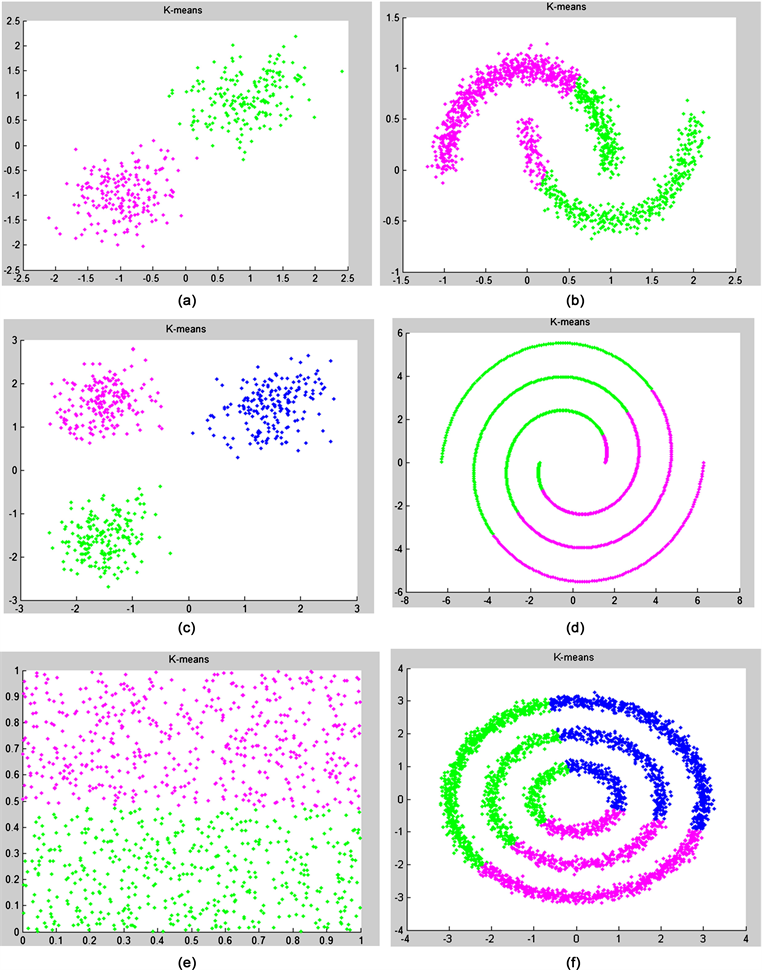Figure 2. K-Mean clustering result graph of artificial data set: (a) Two-cluster; (b) Two moons; (c) Spiral; (d) Three-circles; (e) Checker-board; (f) Three-cluster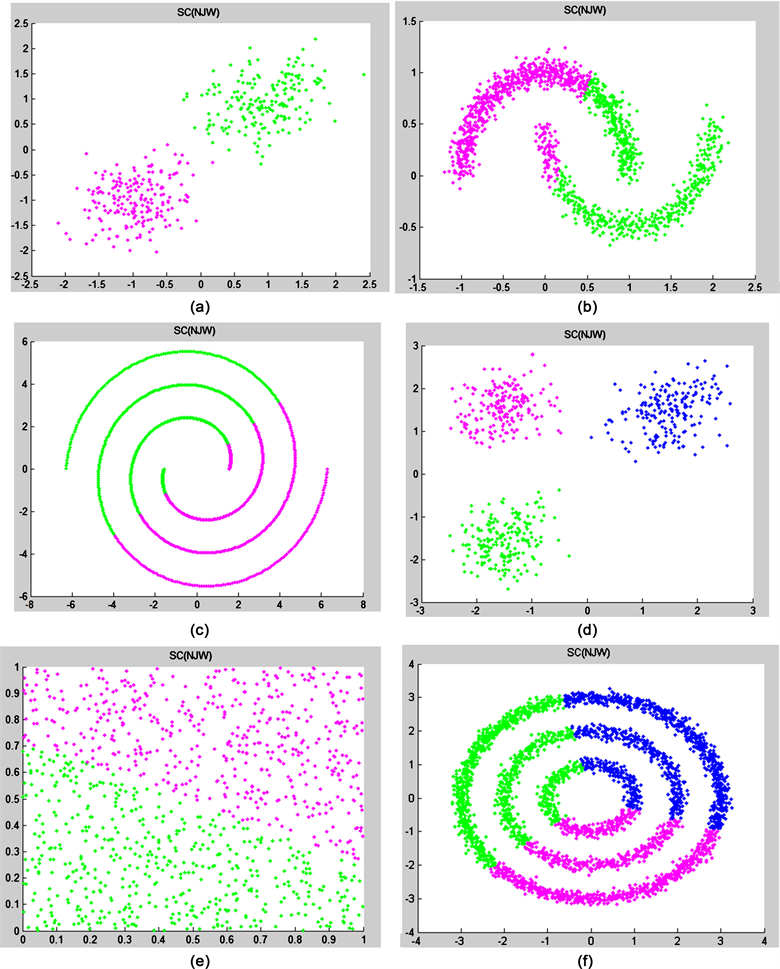Figure 3. Spectral clustering (NJW) result graph of artificial data set: (a) Two-cluster; (b) Two moons; (c) Spiral; (d) Three-circles; (e) Checker-board; (f) Three-cluster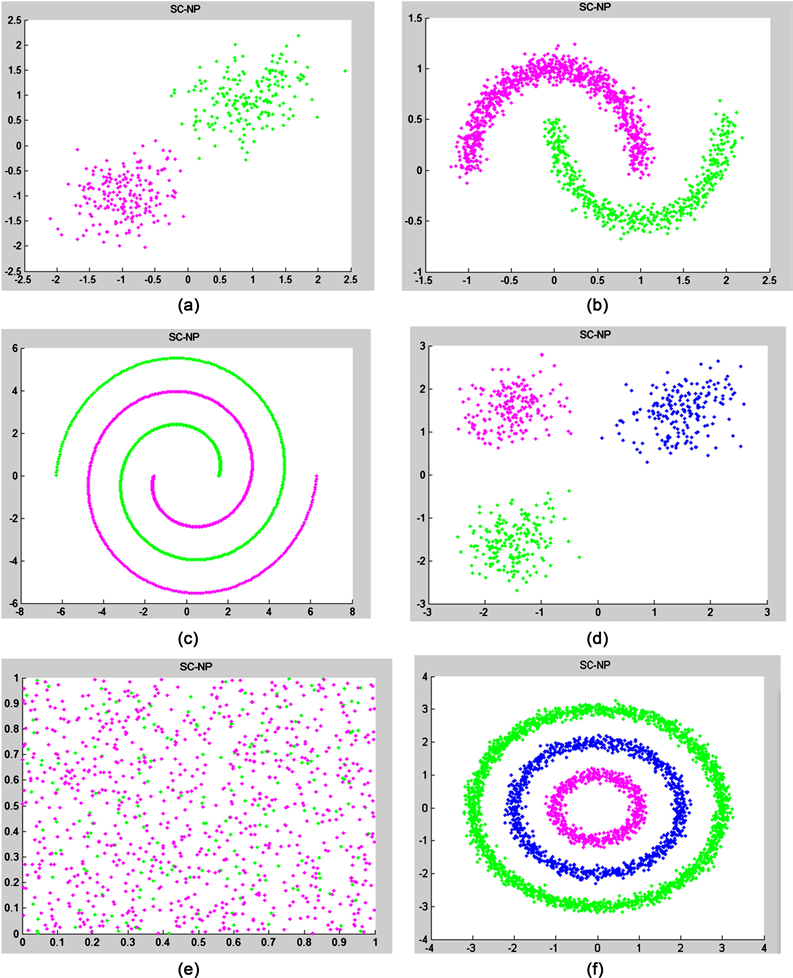Figure 4. NP-SC clustering result graph of artificial data set: (a) Two-cluster; (b) Two moons; (c) Spiral; (d) Three-circles; (e) Checker-board; (f) Three-cluster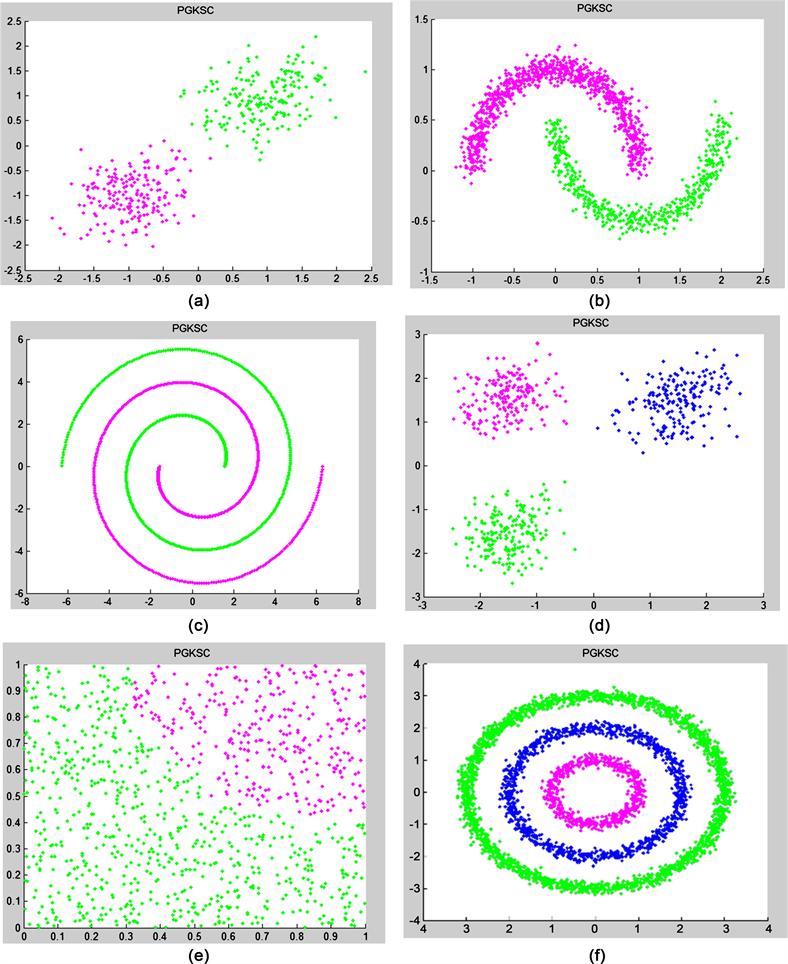Figure 5. PGK-SC clustering result graph of artificial data set: (a) Two-cluster; (b) Two moons; (c) Spiral; (d) Three-circles; (e) Checker-board; (f) Three-cluster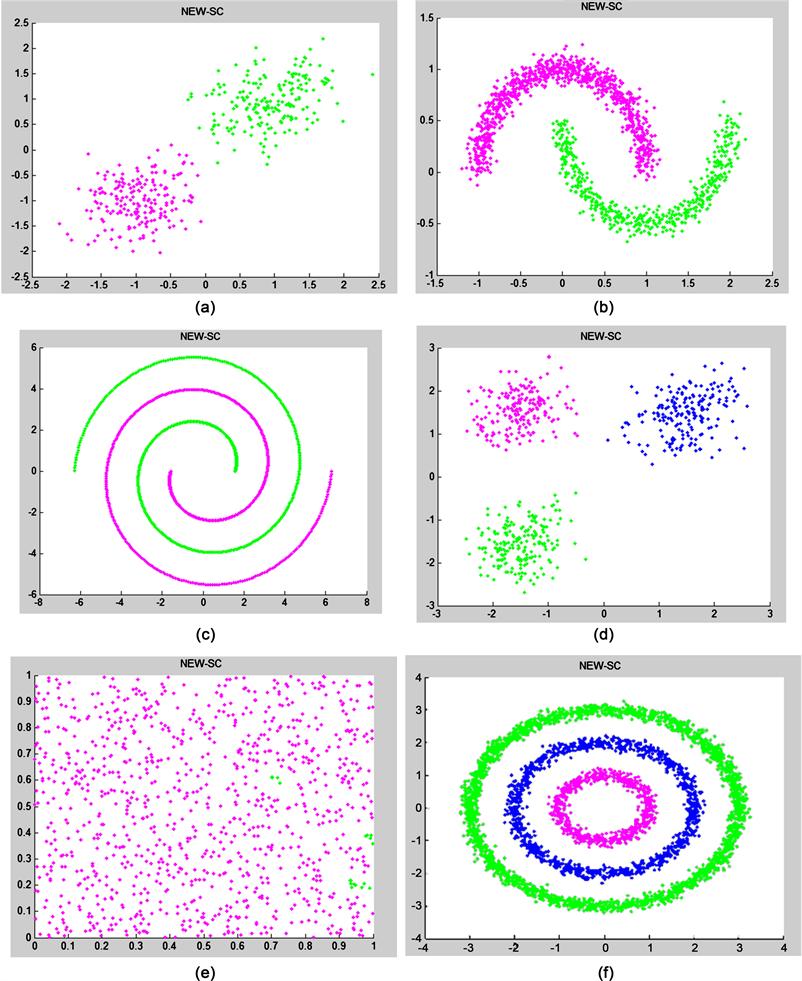Figure 6. NEW-SC clustering result graph of artificial data set: (a) Two-cluster; (b) Two moons; (c) Spiral; (d) Three-circles; (e) Checker-board; (f) Three-clusterTable 2. Accuracy rates of artificial data sets (ACC)Figure 7. Accuracy histogram of artificial data sets

4.4. UCI数据集的实验结果Table 3. Accuracy rates of UCI data set (ACC)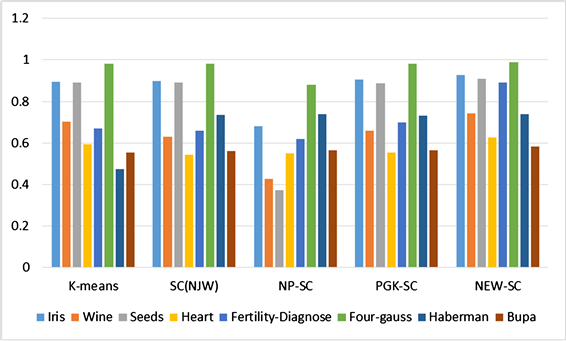Figure 8. Accuracy histogram of UCI data sets

5. 实验结论

  Jain, A.K., Murty, M.N. and Flynn, P.J. (1999) Data Clustering: A Review. ACM Computing Surveys, 31, 264-323. https://doi.org/10.1145/331499.331504  王千, 王成, 冯振元, 等. K-means聚类算法研究综述[J]. 电子设计工程, 2012(4).  孙伟. 谱聚类算法的改进研究[D]: [硕士学位论文]. 兰州: 兰州商学院, 2012.  Cai, D., He, X., Han, J. and Member, S. (2005) Document Clustering Using Locality Preserving Indexing. IEEE Transactions on Knowledge and Data Engineer-ing, 17, 1624-1637. https://doi.org/10.1109/TKDE.2005.198  蔡晓研, 戴冠中, 杨黎斌. 谱聚类算法综述[J]. 计算机科学, 2008, 35(7): 14-18.  von Luxburg, U. (2007) A Tutorial on Spectral Clustering. Statistics and Computing, 17, 395-416. https://doi.org/10.1007/s11222-007-9033-z  Filipponea, M., Camastrab, F., Masullia, F. and Rovetta, S. (2008) A Survey of Kernel and Spectral Methods for Clustering. Pattern Recognition, 41, 176-190. https://doi.org/10.1016/j.patcog.2007.05.018  Nascimento, M.C.V. and de Carvalho, A.C.P.L.F. (2011) Spectral Methods for Graph Clustering—A Survey. European Journal of Operational Research, 211, 221-231. https://doi.org/10.1016/j.ejor.2010.08.012  Chen, W. and Feng, G. (2012) Spectral Clustering: A Semi-Supervised Approach. Neurocomputing, 77, 229-242. https://doi.org/10.1016/j.neucom.2011.09.002  Ozertem, U., Erdogmus, D. and Jenssen, R. (2008) Mean Shift Spectral Clus-tering. Pattern Recognition, 41, 1924-1938. https://doi.org/10.1016/j.patcog.2007.09.009  Donath, W.E. and Hoffman, A.J. (1973) Lower Bounds for the Partitioning of Graphs. IBM Journal of Research and Development, 17, 420-425. https://doi.org/10.1147/rd.175.0420  Fiedler, M. (1973) Algebraic Connectivity of Graphs. Czechoslovak Mathematical Journal, 23, 298-305.  Li, X.-Y. and Guo, L.-J. (2012) Con-structing Affinity Matrix in Spectral Clustering Based on Neighbor Propagation. Neurocomputing, 97, 125-130. https://doi.org/10.1016/j.neucom.2012.06.023  Nataliani, Y. and Yang, M.-S. (2017) Powered Gaussian Kernel Spectral Clustering. Neural Computing and Applications, 31, 557-572. https://doi.org/10.1007/s00521-017-3036-2  Gong, Y.C. and Chen, C. (2008) Locality Spectral Clutering. Proceeding of the 21st Australasian Joint Conference on Artificial Intelligence: Advance in Artificial Intelligence, Springer-Verlag, 348-354. https://doi.org/10.1007/978-3-540-89378-3_34  Shi, J. and Malik, J. (2000) Normalized Cuts and Image Segmentation. IEEE Transactions on Pattern Analysis and Machine Intelligence, 22, 888-905. https://doi.org/10.1109/34.868688  Dhillon, I.S., Guan, Y. and Kulis, B. (2004) Kernel K-Means: Spectral Clustering and Normalized Cuts. In: Proceedings of the 10th ACM SIGKDD International Conference on Knowledge Discovery and Data Mining, ACM, New York, 551-556. https://doi.org/10.1145/1014052.1014118  Ding, C., He, X., Zha, H.-Y., Gu, M. and Simon, H.D. (2001) A Min-Max Cut Algorithm for Graph Partitioning and Data Clustering. Proceedings of the 2001 IEEE International Conference on Data Mining, San Jose, CA, 29 November-2 December 2001, 107-114. https://doi.org/10.1109/ICDM.2001.989507  Hagen, L. and Kahng, A.B. (1992) New Spectral Methods for Ratio Cut Partitioning and Clustering. IEEE Transactions on Computer-Aided Design of Inte-grated Circuits and Systems, 11, 1074-1085. https://doi.org/10.1109/43.159993  McQueen, J. (1967) Some Methods for Classification and Analysis of Multivariate Observations. Proceedings of 5-th Berkeley Symposium on Mathematical Statistics and Probability, 1, 281-297.  Ng, A.Y., Jordan, M.I. and Weiss, Y. (2002) On Spectral Clustering: Analysis and an Algorithm. In: Dietterich, T.G., Becker, S. and Ghahramani, Z., Eds., Proceedings of the 14th Advances in Neural Information Processing Systems, MIT Press, Cambridge, MA, 849-856.